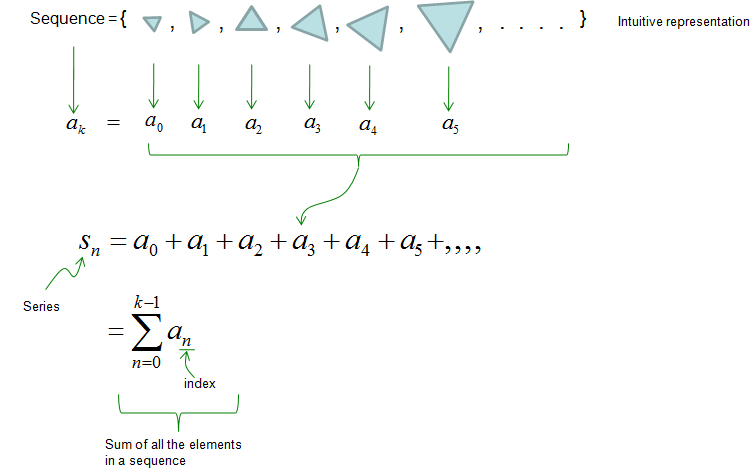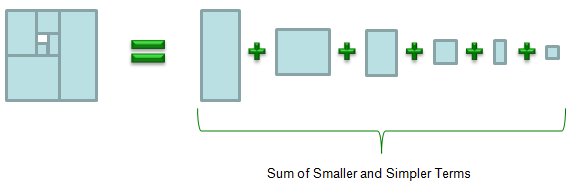Engineering Math - Quick Reference                                 Home : www.sharetechnote.com Series   Mathematical definition of 'Series' is 'sum of all the terms in a sequence'. (It means that you need to have understanding of the concept of sequence first). Is it too dry definition ? Here goes my own definition which I hope is more practical. It may not be very accurate in pure mathematical aspects but I hope it would sound more familiar and less scary -:)   According to my definition, a Serieas is a mathematical tool to split a complicated mathematical object (function) into multiple (in most case, infinite number of ) simpler objects (functions) a mathematical tool to approximate a function (usually complicated function) with sum of simpler functions.   Going back to the formal mathematical definition, the definition of a seriese can be illustrated as shown below. As you see..  when you are given a sequence, just take the summation of all the elements within the sequence, it becomes as Series.Following is a visualization of my definition of a Series. If you look at the object on the left hand side, you see an object that vaguely look like a rectangle. But if you take a closer look you would see some complicated structure in it. On the right side, you would see multiple rectangles and each of the rectangle is in simple shape. But combining all those simple rectangles on the right side, you can build a complicated object as shown on the left side.Most of the Series widely used in engineering area is more like my definition. For example, Taylor serieas is a tool to approximate any function (usually complicated) with sum of multiple simple function called power function. As another example, you can think of Fourier Seriese. Fourier Series a tool to approximate any function (usually complicated) with sum of multiple simple function called 'sin' and/or 'cos'. (I will talk in more detail about Taylor Series and Fourier Series in separate posts later).